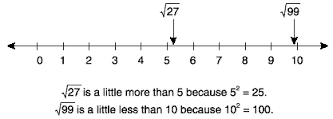## Pre-Algebra Tutorial

#### Intro

In this very large world full of numbers, it can be confusing as to what the difference between a “rational” number and an “irrational” number are. To put it as simple as possible, rational numbers can be whole numbers (1,2,3), integers (-1, -2, -3) and even fractions or decimals (1/4, .1, 2/3). An irrational number is one that runs on without repeating, much like the famous pi symbol.It is also important to understand where the numbers fall, even if they are irrational. For example: the square root of 10 must fall between 3 and 4 on the number line. How did I come to this? Well the square root of 9 is 3 and the square root of 16 is 4. Since 10 is closer to 9 than 16, the square root would also come closer to 3 than it would 4. Another example below:It is also important to understand that irrational numbers can be simplified like fractions. For example, we know that (8/4)=2 and (3/3)=1. So how can we simplify the square root of 8? We know that 8 is not a perfect square root and it falls between 2 and 3 since the closest pairs of perfect roots are 4 and 9.Lets check on a calculator: 2.828427…

#### Sample Problem

Lets start off basic:

1. Is the number 4:
a. whole number
b. integer
c. rational number
d. all of the above

2. Is the square root of 2:
a. rational number
b. irrational number
c. integer
d. none of the above

3. 0.5 and -2 are rational numbers:
A. true
B. false

Solve where to find the numbers:

4. The square root of 11 falls between:
a. 0 and 1
b. 1 and 2
c. 2 and 3
d. 3 and 4

5. The square root of 27:
a. Is 9
b. Is 3
c. falls between 5 and 6
d. falls between 8 and 9

6. The simplified version of the square root of 54:
a. 3 square roots of 6
b. 9 square roots of 6
c. 6 square roots of 9
d. 5 square roots of 4

#### Solution

1. d
If we look back at the graph, the number 4 falls in the very middle circle making it a whole number, an integer, and a rational number.

2. b
The square root of 2 equals 1.4142135… and will continue on forever without repeating, making it an irrational number.

3. true
Both 0.5 and -2 are rational because they do not repeat forever.

4. d
9 is a perfect root of 3 and 16 is a perfect root of 4. Since 11 is between 9 and 16, it will fall between 3 and 4.

5. c
The closest perfect square roots to the number 27 are 25 and 36. Since the square root of 25 is 5 and the square root of 36 is 6, the square root of 27 must fall between 5 and 6 on the number line.

6. a
The square root of 54 is equal to the square root of 9×6. We then can take the square root of 9 and multiply it by the square root of 6. The square root of 9 is 3, but the square root of 6 cannot be simplified any further. Therefore, 3 square roots of 6 is equal to the square root of 54 or 7.34846…